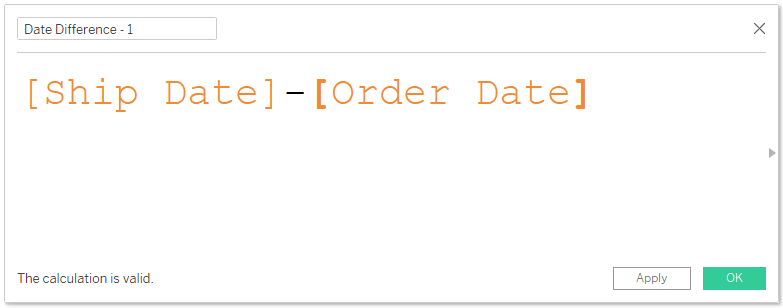Search
• Bernard

# DATEDIFF() Function in Tableau

Updated: May 17DATEDIFF() function is used to compute difference between two dates in Tableau (Date1 & Date2). In this article, I would like to share with you how you can apply this function in your Tableau assignments. But before that, lets first explore a different approach for computing difference between dates.

### Date difference – Simple Arithmetic

Using Superstores data set, I can compute the number of days it takes to ship products in different Regions’ using the following arithmetic calculation.Opening my data view reveals that this computation is returning the difference between the two dates at day level – which is what am expecting.Using this calculation to answer my questions, reveals that the average time it takes to ship products is approximately 4 days in all the regions.(Although this is a simple formula to implement, it presents one constrain, date difference is computed at day level by default – hence you can’t use this simple arithmetic to compute difference using other date parts such as month, quarter, week etc.)

### DATEDIFF() Function to the rescue

Before applying this function, lets first look at the syntax

DATEDIFF(date_part, date1, date2, [Start_of_week])

This calculation gives you more control over how date differences are computed in Tableau. Using this function, you have the freedom to specify the date part you would like to use in your computation e.g. month, day, quarter, year etc. or even use a parameter to dynamically change your data parts. While at the same time you can specify which day to use as your week start.

Let’s recompute our problem again but now using DATEDIFF() function;Using this calculation to answer our problem.(The results of the two date difference computations are not different; however, the second calculation gives you more control as a developer – helping you create more user centered visualizations)

### Bonus point

You can always use parameters to enable users change the date part dynamically as they would like. In this case, have create a parameter field consisting of the following date parts.You can use this parameter in your calculation using this formulaUsing the above calculation will always compute date difference as per the user’s current selection. See the results when user selects ‘day’ and ‘month’ as data parts.I hope this was helpful to you. To receive more of the Tableau tips and tricks, kindly subscribe to our mailing list below.